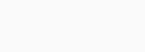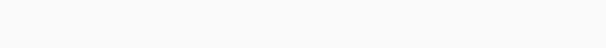# Apriori Algorithm in Python

Hey guys!! In this tutorial, we will learn about apriori algorithm and its implementation in Python with an easy example.

## What is Apriori algorithm?

Apriori algorithm is a classic example to implement association rule mining. Now, what is an association rule mining? Association rule mining is a technique to identify the frequent patterns and the correlation between the items present in a dataset.

For example, say, there’s a general store and the manager of the store notices that most of the customers who buy chips, also buy cola. After finding this pattern, the manager arranges chips and cola together and sees an increase in sales. This process is called association rule mining.

More information on Apriori algorithm can be found here: Introduction to Apriori algorithm

## Working of Apriori algorithm

Apriori states that any subset of a frequent itemset must be frequent.
For example, if a transaction contains {milk, bread, butter}, then it should also contain {bread, butter}. That means, if {milk, bread, butter} is frequent, then {bread, butter} should also be frequent.

The output of the apriori algorithm is the generation of association rules. This can be done by using some measures called support, confidence and lift. Now let’s understand each term.

Support: It is calculated by dividing the number of transactions having the item by the total number of transactions.

Confidence: It is the measure of trustworthiness and can be calculated using the below formula.
Conf(A => B)=Lift: It is the probability of purchasing B when A is sold. It can be calculated by using the below formula.
Lift(A => B)=1. Lift(A => B) =1 : There is no relation between A and B.
2. Lift(A => B)> 1: There is a positive relation between the item set . It means, when product A is bought, it is more likely that B is also bought.
3. Lift(A => B)< 1: There is a negative relation between the items. It means, if product A is bought, it is less likely that B is also bought.

Now let us understand the working of the apriori algorithm using market basket analysis.
Consider the following dataset:

Transaction ID                             Items
T3                                   Milk
T4                                   Cola
T5                                   Chips, Cola, Milk
T6                                   Chips, Cola, Milk

Step 1:
A candidate table is generated which has two columns: Item and Support_count. Support_count is the number of times an item is repeated in all the transactions.
Item                            Support_count
Chips                                    4
Cola                                      4
Milk                                      5
Given, min_support_count =3. [Note: The min_support_count is often given in the problem statement]

Step 2:
Now, eliminate the items that have Support_count less than the min_support_count. This is the first frequent item set.
Item                            Support_count
Chips                                    4
Cola                                      4
Milk                                      5

Step 3:
Make all the possible pairs from the frequent itemset generated in the second step. This is the second candidate table.
Item                            Support_count
{Chips, Cola}                                  3
{Chips, Milk }                                 3
{Cola, Milk}                                    3
[Note: Here Support_count represents the number of times both items were purchased in the same transaction.]

Step 4:
Eliminate the set with Support_count less than the min_support_count. This is the second frequent item set.
Item                            Support_count
{Chips, Cola}                                  3
{Chips, Milk }                                 3
{Cola, Milk}                                    3

Step 5:
Now, make sets of three items bought together from the above item set.
Item                                     Support_count
{Chips, Cola, Milk}                         3

Since there are no other sets to pair, this is the final frequent item set. Now to generate association rules, we use confidence.
Conf({Chips,Milk}=>{Cola})== 3/3 =1
Conf({Cola,Milk}=>{Chips})= 1
Conf({Chips,Cola}=>{Chips})= 1

The set with the highest confidence would be the final association rule. Since all the sets have the same confidence, it means that, if any two items of the set are purchased, then the third one is also purchased for sure.

## Implementing Apriori algorithm in Python

Problem Statement:
The manager of a store is trying to find, which items are bought together the most, out of the given 7.
Below is the given datasetDataset

Before getting into implementation, we need to install a package called ‘apyori’ in the command prompt.

`pip install apyori`1. Importing the libraries3. Display the data4. Generating the apriori model5. Display the final rulesThe final rule shows that confidence of the rule is 0.846, it means that out of all transactions that contain ‘Butter’ and ‘Nutella’, 84.6% contains ‘Jam’ too.
The lift of 1.24 tells us that ‘Jam’ is 1.24 times likely to be bought by customers who bought ‘Butter’ and ‘Nutella’ compared to the customers who bought ‘Jam’ separately.

This is how we can implement apriori algorithm in Python.